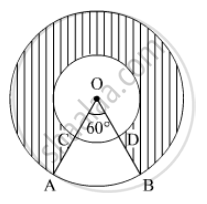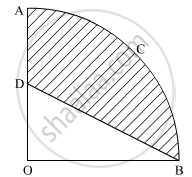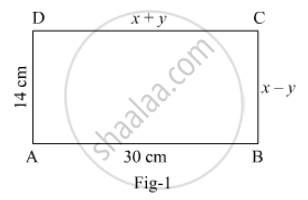Share

# Important Questions for CBSE Class 10 - CBSE - Mathematics

Course
Subjects
Topics
Subjects
Popular subjects
Topics
Mathematics
< prev 1 to 20 of 765 next >

Prove that the lengths of the tangents drawn from an external point to a circle are equal.

Appears in 20 question papers
Chapter: [3.01] Circles
Concept: Number of Tangents from a Point on a Circle

Prove that the tangent at any point of a circle is perpendicular to the radius through the point of contact.

Appears in 14 question papers
Chapter: [3.01] Circles
Concept: Tangent to a Circle

Prove that a parallelogram circumscribing a circle is a rhombus.

Appears in 8 question papers
Chapter: [3.01] Circles
Concept: Number of Tangents from a Point on a Circle

Prove that the tangents drawn at the ends of a diameter of a circle are parallel.

Appears in 5 question papers
Chapter: [3.01] Circles
Concept: Number of Tangents from a Point on a Circle

In an AP of 50 terms, the sum of first 10 terms is 210 and the sum of its last 15 terms is 2565. Find the A.P.

Appears in 4 question papers
Chapter: [2.02] Arithmetic Progressions
Concept: Arithmetic Progression

Solve the following quadratic equation for x: 4x^2 + 4bx – (a^2 – b^2) = 0

Appears in 4 question papers
Concept: Solutions of Quadratic Equations by Completing the Square

Prove that opposite sides of a quadrilateral circumscribing a circle subtend supplementary angles at the centre of the circle.

Appears in 4 question papers
Chapter: [3.01] Circles
Concept: Number of Tangents from a Point on a Circle

Construct a triangle ABC with sides BC = 7 cm, ∠B = 45° and ∠A = 105°. Then construct a triangle whose sides are 3/4 times the corresponding sides of ∆ABC.

Appears in 4 question papers
Chapter: [3.02] Triangles
Concept: Application of Pythagoras Theorem in Acute Angle and Obtuse Angle

A bucket open at the top is in the form of a frustum of a cone with a capacity of 12308.8 cm3. The radii of the top and bottom circular ends are 20 cm and 12 cm, respectively. Find the height of the bucket and the area of metal sheet used in making the bucket. (use π = 3.14)

Appears in 4 question papers
Chapter: [4.01] Heights and Distances
Concept: Heights and Distances

In Figure , two concentric circles with centre O, have radii 21cm and 42 cm. If ∠ AOB = 60°, find the area of the shaded region. [use π=22/7]Appears in 4 question papers
Chapter: [7.01] Areas Related to Circles
Concept: Problems Based on Areas and Perimeter Or Circumference of Circle, Sector and Segment of a Circle

In the given figure, OACB is a quadrant of a circle with centre O and radius 3.5 cm. If OD = 2 cm, find the area of the shaded region.Appears in 4 question papers
Chapter: [7.01] Areas Related to Circles
Concept: Areas of Sector and Segment of a Circle

What is the HCF of the smallest prime number and the smallest composite number?

Appears in 3 question papers
Chapter: [1.01] Real Numbers
Concept: Fundamental Theorem of Arithmetic

Given that sqrt2 is irrational prove that (5 + 3sqrt2)  is an irrational number

Appears in 3 question papers
Chapter: [1.01] Real Numbers
Concept: Revisiting Irrational Numbers

Find HCF and LCM of 404 and 96 and verify that HCF × LCM = Product of the two given numbers.

Appears in 3 question papers
Chapter: [1.01] Real Numbers
Concept: Fundamental Theorem of Arithmetic

Speed of a boat in still water is 15 km/h. It goes 30 km upstream and returns back at the same point in 4 hours 30 minutes. Find the speed of the stream.

Appears in 3 question papers
Chapter: [2.01] Pair of Linear Equations in Two Variables
Concept: Pair of Linear Equations in Two Variables

In Fig. 1, ABCD is a rectangle. Find the value of x and y.Appears in 3 question papers
Chapter: [2.01] Pair of Linear Equations in Two Variables
Concept: Equations Reducible to a Pair of Linear Equations in Two Variables

In an A.P., if S5 + S7 = 167 and S10=235, then find the A.P., where Sn denotes the sum of its first n terms.

Appears in 3 question papers
Chapter: [2.02] Arithmetic Progressions
Concept: Sum of First n Terms of an AP

Find the 9th term from the end (towards the first term) of the A.P. 5, 9, 13, ...., 185

Appears in 3 question papers
Chapter: [2.02] Arithmetic Progressions
Concept: Sum of First n Terms of an AP

If the sum of first 7 terms of an A.P. is 49 and that of its first 17 terms is 289, find the sum of first n terms of the A.P.

Appears in 3 question papers
Chapter: [2.02] Arithmetic Progressions
Concept: Sum of First n Terms of an AP

The first three terms of an AP respectively are 3y – 1, 3y + 5 and 5y + 1. Then y equals:

(A) –3
(B) 4
(C) 5
(D) 2

Appears in 3 question papers
Chapter: [2.02] Arithmetic Progressions
Concept: Arithmetic Progression
< prev 1 to 20 of 765 next >
Important Questions for CBSE Class 10 CBSE Mathematics. You can further filter Important Questions by subjects and topics. Chapter wise important Questions for Class 10 CBSE. it gets easy to find all Class 10 important questions with answers in a single place for students. Saving time and can then focus on their studies and practice. Important questions for Class 10 chapter wise with solutions

Class 10 Mathematics can be very challenging and complicated. But with the right ideas and support, you will be able to make it work. That’s why we have the class 10 Mathematics important questions with answers pdf ready for you. All you need is to acquire the PDF with all the content and then start preparing for the exam. It really helps and it can bring in front an amazing experience every time if you're tackling it at the highest possible level.